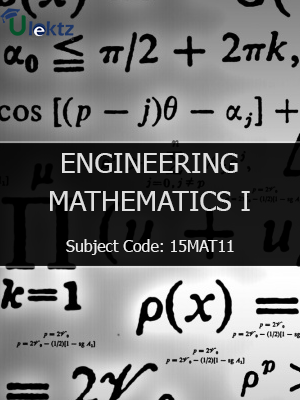•My WalletMy Order
•My Profile
•My Connections
•My Books
•My Videos
•My Tests
•My Calender
•My Messages
•My Shopping Cart
•My Orders
•Account Settings
•Help

# Book Details# Engineering Mathematics - I

 Course Code : 15MAT11 Author : uLektz University : Visvesvaraya Technological University, Karnataka (VTU) Regulation : 2015 Categories : Civil Format :ePUB3 (DRM Protected) Type : eBook

FREE

Description :Engineering Mathematics - I of 15MAT11 covers the latest syllabus prescribed by Visvesvaraya Technological University, Karnataka (VTU) for regulation 2015. Author: uLektz, Published by uLektz Learning Solutions Private Limited.

Note : No printed book. Only ebook. Access eBook using uLektz apps for Android, iOS and Windows Desktop PC.

##### Topics
###### Module 1 Differential Calculus -1:

1.1 Determination of nth order derivatives of Standard functions - Problems.

1.2 Leibnitz’s theorem (without proof) - problems.

1.3 Polar Curves -Angle between the radius vector and tangent, angle between two curves, Pedal equation of polar curves.

1.4 Derivative of arc length -Cartesian, Parametric and Polar forms (without proof) - problems.

1.5 Curvature and Radius of Curvature-Cartesian, Parametric, Polar and Pedal forms (without proof) -problems

###### Module 2 Differential Calculus -2

2.1 Taylor’s and Maclaurin’s theorems for function of one variable(statement only)- problems.

2.2 Evaluation of Indeterminate forms.

2.3 Partial derivatives -Definition and simple problems, Euler’s theorem(without proof) – problems

2.4 Total derivatives, partial differentiation of composite functions-problems.

2.5 Definition and evaluation of Jacobians.

###### Module 3 Vector Calculus:

3.1 Derivative of vector valued functions, Velocity, Acceleration and related problems, Scalar and Vector point functions.

3.2 Definition of Gradient, Divergence and Curl-problems-Solenoidal and Irrotational vector fields. Vector identities - div(ɸA), curl (ɸA ), curl( grad ɸ), div(curl A).

###### Module 4 Integral Calculus:

4.2 Differential Equations-Solution of first order and first degree differential equations

4.3 Exact, reducible to exact and Bernoulli’s differential equations-Orthogonal trajectories in Cartesian and polar form. Simple problems on Newton's law of cooling.

###### Module 5 Linear Algebra

5.1 Rank of a matrix by elementary transformations, solution of system of linear equations

5.2 Gauss-elimination method, Gauss –Jordan method and Gauss-Seidel method

5.3 Eigen values and Eigen vectors, Rayleigh’s power method to find the largest Eigen value and the corresponding Eigen vector.

5.4 Linear transformation, diagonalisation of a square matrix . Reduction of Quadratic form to Canonical form

### Related Books